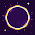### leetcode Question115: Unique Binary Search Trees II

Unique Binary Search Trees II

Given n, how many structurally unique BST's (binary search trees) that store values 1...n?
For example,
Given n = 3, there are a total of 5 unique BST's.
   1         3     3      2      1
\       /     /      / \      \
3     2     1      1   3      2
/     /       \                 \
2     1         2                 3

Analysis:

The basic idea is still using the DFS scheme. It is a little hard to think the structure of the argument list in the function. It is clear that for each tree/subtree, we will set the root as the start position to the end position, and recursively construct the left subtree with the left part and the right subtree with the right part.
So first we can have
void dfs (int st, int ed     ){
if (st>ed) {   // generate a null node }
else{
for (int i=st;i<=ed;i++){

dfs(st,i-1,   );     //generate left subtree
dfs(i+1,ed,   );  // generate right subtree
}
}

}

Next step is to think about how to store all the possible solutions.
This is important ! Think about the root node, all the possible solutions of the tree are from the combinations of all the possible solutions of its left subtree, and its right subtree. One step further, if we have a root node and a left node, for the left node, still the subtrees below it are the combinations of the possible solutions of its left and right subtree, until the leaf node.

In other words, we store all the possible solutions for each node, instead of storing the only tree. So, we can have
void dfs(int st, int ed, vector<TreeNode *> res){}
in this function, recursively generate the left tree and right tree, then construct the current node, and push it to the current solution vector.
Details see the code.

Code:
/**
* Definition for binary tree
* struct TreeNode {
*     int val;
*     TreeNode *left;
*     TreeNode *right;
*     TreeNode(int x) : val(x), left(NULL), right(NULL) {}
* };
*/
class Solution {
public:
void dfs(int st,int ed,vector<TreeNode *> &res){
if (st>ed){
res.push_back(NULL);
}else{
for (int i=st;i<=ed;i++){
vector<TreeNode *> lefts;
dfs(st,i-1,lefts);
vector<TreeNode *> rights;
dfs(i+1,ed,rights);

for (int li = 0; li<lefts.size();li++) {
for (int ri =0; ri<rights.size();ri++){
TreeNode* node = new TreeNode(i);
node->left=lefts[li];
node->right=rights[ri];
res.push_back(node);
}
}
}
}
}

vector<TreeNode *> generateTrees(int n) {
// Start typing your C/C++ solution below
// DO NOT write int main() function
vector<TreeNode*> res;
dfs(1,n,res);
return res;
}
};


1.2.